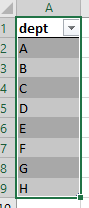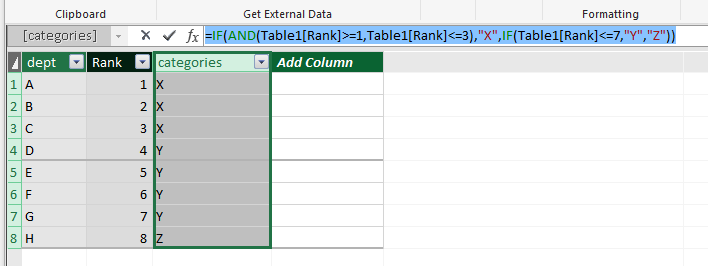cancel
Showing results for
Did you mean:Regular Visitor

## powerpivot OR function limited

I get the message "The end of the input was reached". What I'm trying to do is have multiple 'OR'-functions in my table. I've tried || , but that didn't help much.

What I need is;

column [dept] has 8 unique values(A, B, C, D, E, F, G, H),  which I want to categorize in 3 categories X, Y and Z. For example, A through C go in X, D through F go in Y, what's left in Z.

How would I handle this in Powerpivot?

1 ACCEPTED SOLUTIONMicrosoft

Hi @Jawroy,

I try to reproduce your scenario and get expected result as follows.2. Create a rank calculated column using the formula.

`=RANKX(Table1,Table1[dept],,ASC)`

3. Based on rank column value, calculate the expected result using formula.

`=IF(AND(Table1[Rank]>=1,Table1[Rank]<=3),"X",IF(Table1[Rank]<=7,"Y","Z"))`

Please see the result shown in following screenshot.If you have other issues, don't hesitate to let me know.

Best Regards,
Angelia

2 REPLIES 2Microsoft

Hi @Jawroy,

I try to reproduce your scenario and get expected result as follows.2. Create a rank calculated column using the formula.

`=RANKX(Table1,Table1[dept],,ASC)`

3. Based on rank column value, calculate the expected result using formula.

`=IF(AND(Table1[Rank]>=1,Table1[Rank]<=3),"X",IF(Table1[Rank]<=7,"Y","Z"))`

Please see the result shown in following screenshot.If you have other issues, don't hesitate to let me know.

Best Regards,
AngeliaRegular Visitor

Thanks Angelia